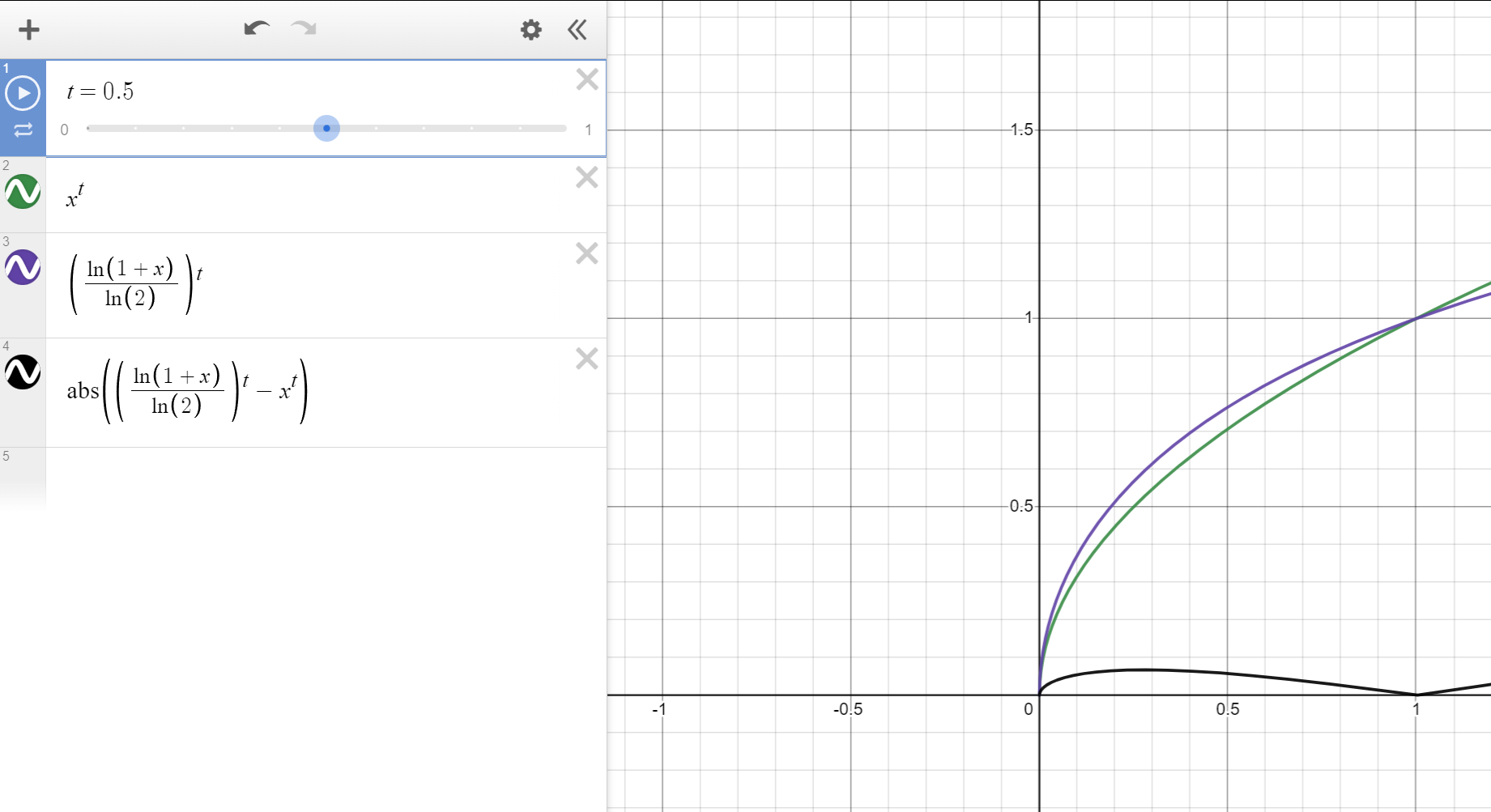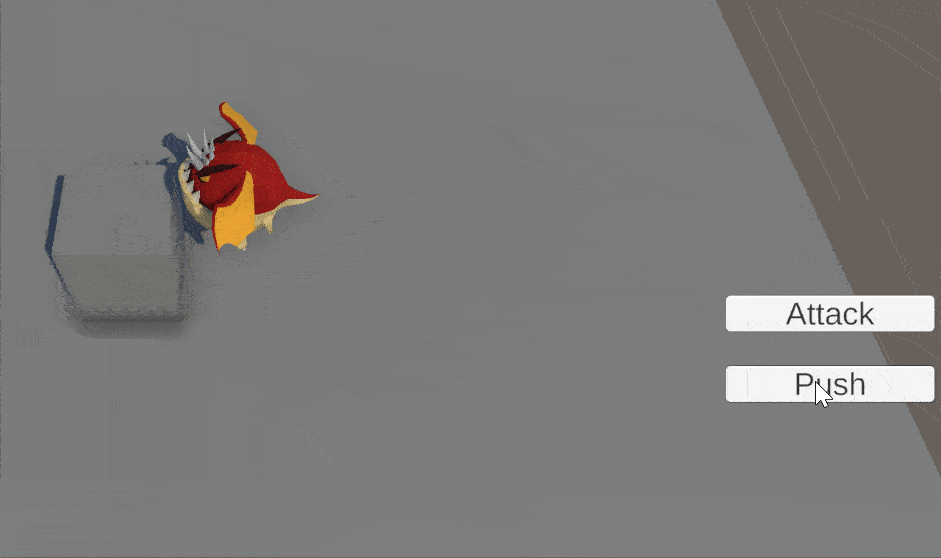# Implementing a parametric pushback in Unity

Published

In a previous article, we implemented a recoil effect in Unity. Now we’ll improve on our previous code to allow for a smooth pushback effect dependent on duration and distance. The idea is to replicate an effect similar to Final Fantasy Tactics Advance: when a unit receives a critical attack, it gets pushed back by 2 cells.

So how are we going to do that? We’ll use some quick maths. The main idea is that we are lerping between an initial position and a target position depending on time elapsed and duration of the animation.

``````float time_pct = time_elapsed / duration;
Vector3 curr_pos = Vector3.Lerp(initial_pos, target_pos, time_pct);``````

Now the main issue with the previous code is that this is linear interpolation so the unit gets moved at a constant speed and suddenly stops, while pushback / recoil usually has a decreasing force.

To give a more realistic impression, we’ll want to remap from time_pct to another value using a function that exhibits decay. Visually, this would mean a function that takes a value from [0; 1] and remaps it to [0; 1], but would have fast growth at first then flattens while approaching x = 1.0.

It’s a common approach to use a tool such as AnimationCurve to easily remap an input to get this effect. It’s a simple approach, and it works, but in my opinion it lacks some flexility if you need to handle different cases or want to iterate quickly: maybe you’re unsure of the target duration, or how strong the decay should be. Using a mathematical function instead of an AnimationCurve can help you get the level of flexibility you need.

So which functions should we use? As described previously, we need a function that can take an input from [0; 1] and output a value to [0; 1] while exhibiting a curved pattern. There are a number of functions that you can use but you may have to adjust them a bit to fit your needs, while some don’t need much work. For example, with x in [0; 1] you can use Mathf.pow(x, p) with p in [0; 1].

Let’s take a more involved example, to show off how we can adjust a preexisting function: the log function (Mathf.Log10). Now, the log function exhibits this curved aspect that we’re looking for, but its values need to be remapped since ln(0) goes to -inf.
The good thing about it though, is that log(1) = 0 so with “t” the current value of elapsed_time / anim_duration, we can 1.0 to our value of t so that our new function will give 0 for t = 0. Now the next issue is to focus on the other end of the curve: it needs to be equal to 0 if t == 1.0. Since our current function is Mathf.Log10(1f + t), then we can get a value of 1.0 with the following: f(1.0) = Mathf.Log10(1f + 1f) / Mathf.Log10(2) = 1.0.
Finally, as our final value is in the range [0.0; 1.0], we can use a power function on it, like we did on the previous example, to control the slope of the function. Using this, we now have a log10 based function that remaps a value from [0; 1.0] to [0.0; 1.0] while exhibiting our intended behaviour. Congrats!

Let’s visualize the evolution of our function using Desmos. As you can see, the y = ln(1+x)/ln(2) function exhibits some curvature compared to y = x.

As mentionned, you can use other functions as long as they remap into the appropriate range. Let’s also quickly compare our log-based solution to the pow(x, p) method.The log method exhibits a stronger rise in the low end of the curve

So now that we have our function, let’s implement it in Unity. The logic is pretty straightforward, since we’re just lerping between 2 vectors using a function so I’ll just show code wrapped in a Coroutine.

``````using System.Collections;
using UnityEngine;

/// Player controller for dev of fighting
public class CharacterPushback : MonoBehaviour
{
public float duration;
public float power;

public void Push() {
StartCoroutine(Coroutine_Push(init_pos, target_pos));
}

IEnumerator Coroutine_Push(Vector3 initial_pos, Vector3 target_pos) {
float elapsed = 0f;
while (elapsed < duration) {
float lerp_factor = Mathf.Pow(Mathf.Log10(1f + elapsed / duration) / Mathf.Log10(2f), power);
transform.position = Vector3.Lerp(initial_pos, target_pos, lerp_factor);
yield return null;
elapsed += Time.deltaTime;
}

transform.position = target_pos;
}
}``````

Now we’re free to use it in-game! Let’s take a look:Pushing back the dragon 2 units, with a nice, smooth decay

This is all pretty straightforward. Now it’s your call to adjust power and duration to whatever you think is best!